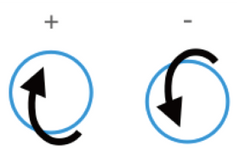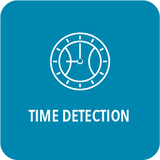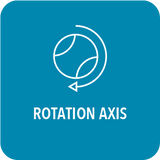# - PICTHER -### AVG. Speed (kph/mph)

Average ball velocity pitched by a pitcher.### Total spin (rpm)

Average RPM of a Pitcher's Pitch.### Top spin (rpm)

Spin rate acting on the horizontal axis and rotating in the vertical direction.

*Positive when rotation is from 6 o'clock to 12 o'clock; negative when rotation is from 12 o'clock to 6 o'clock.### Side spin (rpm)

Spin rate acting on the vertical axis and rotating in the horizontal direction.

*Positive when rotation is from 9 o'clock to 3 o'clock; negative when rotated from 3 o'clock to 9 o'clock.### Release time (s)

The number of seconds it takes from the moment the pitcher starts pitching to the pitcher releases the ball.

### Travel time (s)

The number of seconds it takes from the moment the pitcher starts pitching to the catcher catching the ball.### Vertical break (cm/inch)

The vertical movement of the ball trajectory affected by the rotation speed and the zero rotation speed.

*up is positive; down is negative.### Horizontal break (cm/inch)

The horizontal movement of the ball trajectory affected by the rotation speed and the zero rotation speed trajectory.

*Pitcher's perspective, right is positive; left is negative.### Spin direction (hh:mm/°)

The direction of rotation, expressed in the direction of the clock's hour or in degrees.

When expressed in degrees, the 12 o'clock direction is 0 degrees, and the degrees increase clockwise.

*Shown from pitcher's perspective

### Spin efficiency (%)

The spin ratio that produces effective displacement on the pitch.### Gyro degree (°)

The angle between the rotation axis and the effective rotation plane, usually the smaller the angle, the greater the rotation efficiency.

*The effective rotation plane referred to here is the two-dimensional plane formed by the third base to the first base, the ground to the sky, that is, the plane perpendicular to the pitching direction.

*When the ball is clockwise (the rotation axis is facing forward), the right side is positive; the left side is negative.

*When the ball rotates counterclockwise (the rotation axis is facing backward), the right side is negative; the left side is positive.

# - CATCHER -### Total spin (rpm)

Average RPM of a catcher's pitch.### Pop time (s)

The number of seconds it takes from the moment the catcher catches the ball to the time the ball enters the baseman's glove.

### Exchange time (s)

The number of seconds it takes from the moment the catcher catches the ball to the catcher releases the ball.

### Bounces (#)

Number of bounces on the ground when the catcher throws the ball to the baseman.### Spin direction (hh:mm/°)

The direction of rotation, expressed in the direction of the clock's hour or in degrees.

When expressed in degrees, the 12 o'clock direction is 0 degrees, and the degrees increase clockwise.

*Shown from pitcher's perspective.

### Spin efficiency (%)

The spin ratio that produces effective displacement on the pitch.### Gyro degree (°)

The angle between the rotation axis and the effective rotation plane, usually the smaller the angle, the greater the rotation efficiency.

*The effective rotation plane referred to here is the two-dimensional plane formed by the third base to the first base, the ground to the sky, that is, the plane perpendicular to the pitching direction.

*When the ball is clockwise (the rotation axis is facing forward), the right side is positive; the left side is negative.

*When the ball rotates counterclockwise (the rotation axis is facing backward), the right side is negative; the left side is positive.

--

*Some metrics are not included in the basic functions and need to be combined with a software subscription plan.